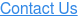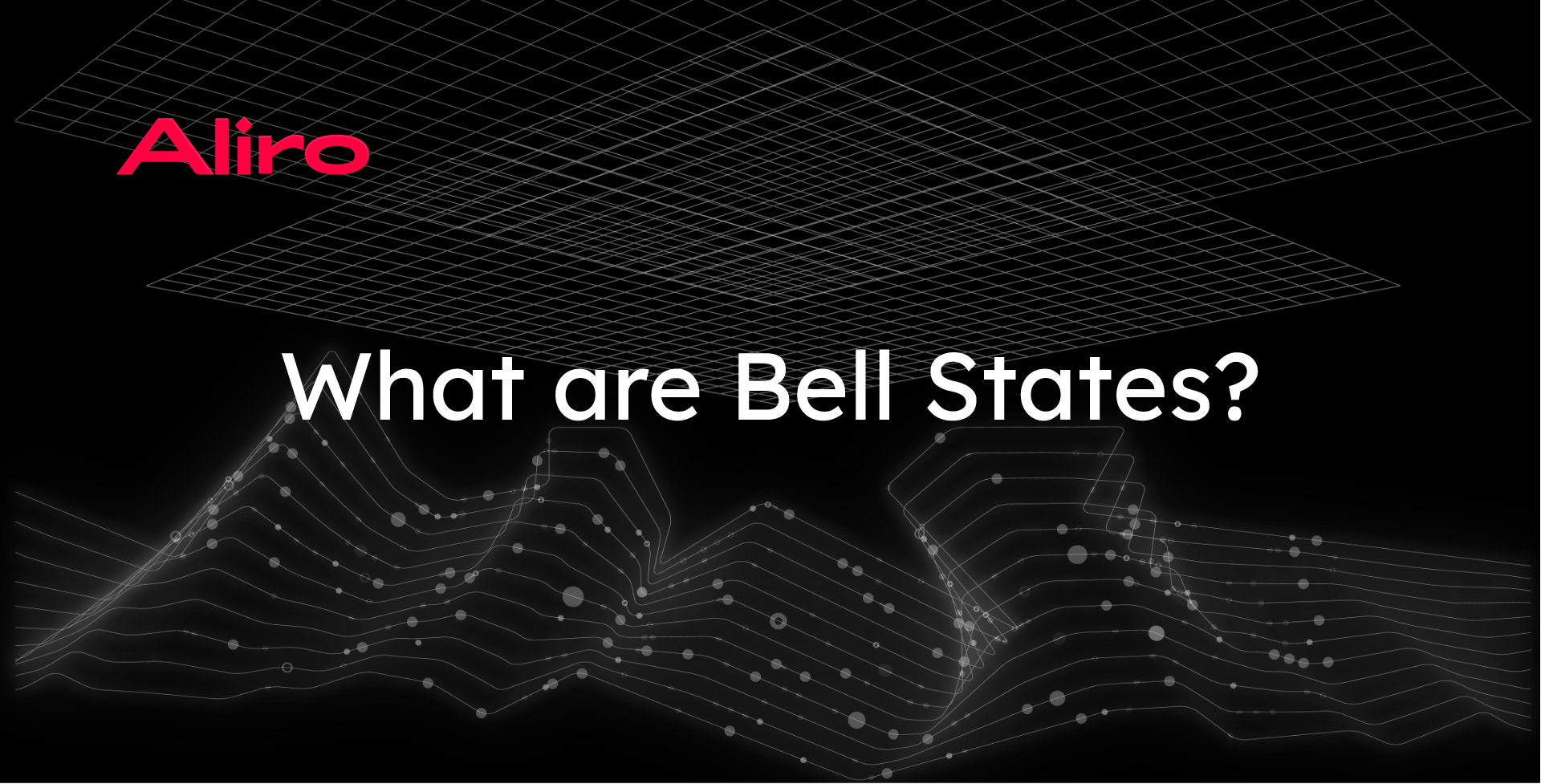# What are Bell States?The basic service provided by classical networks is the transmission of data from sender to receiver. The fundamental service provided by quantum networks, on the other hand, is to generate end-to-end quantum entanglement between endpoints, which can then be consumed by applications. In most quantum networking protocols and algorithms, this entanglement is generated using Bell pairs.

In this post, which assumes no prior knowledge of quantum networks, we will explore what Bell pairs are and some of the quantum networking protocols that make use of them. By better understanding this fundamental resource, readers will acquire insight into quantum networks as a whole.

## History: EPR paradox and origins of Bell pairs

In 1935, physicists Albert Einstein, Boris Podolsky, and Nathan Rosen published a paper containing a thought experiment, now known as the EPR paradox. The EPR paradox describes a situation in quantum mechanics that violates a foundational law of classical physics. This law is known as “locality,” which states that an object cannot influence another object in less time than it takes for light to travel between the two objects.

This violation of locality was used as an argument that quantum mechanics could not be a complete theory. This suggested to Einstein and his colleagues that there must be a classical "hidden-variable" theory underlying quantum mechanics that “fixed” this violation. In the 1960’s, John S. Bell showed this hidden-variable solution was not needed and, in so doing, further revealed the strange properties of quantum entanglement.

Bell reformulated the EPR paradox and demonstrated that no classical hidden-variable theory that kept locality could fully describe quantum mechanics. To do this, he used a specific set of quantum states, now known as “Bell pairs,” which are today the canonical example of “entangled” states.

## Recap: Quantum bits, superposition, and entanglement

Just as classical bits are the fundamental building block of classical computers, quantum bits--or “qubits”--are the basic unit of information in quantum computers. Whereas classical bits can either have a value of 0 or 1, qubits can be in a combination of the states $$\ket{0}$$ and $$\ket{1}$$. If the qubit is not exactly in the state $$\ket{0}$$ or in the state $$\ket{1}$$, but rather in some combination of both the $$\ket{0}$$ and $$\ket{1}$$ states, then we say the qubit is in a “superposition” of the two states.

Measurement: When we measure a qubit that is exactly in the $$\ket{0}$$ state or $$\ket{1}$$ state, then the qubit will remain in that state. However, if we measure a qubit that is in a superposition, then this superposition collapses from being in a combination of the two states to being exactly in one of the two states. We cannot predict if the superposition will collapse into either the $$\ket{0}$$ or the $$\ket{1}$$ state with certainty, but we can know the probabilities of being measured in either of the two states.

Entanglement: It turns out that quantum states can extend over multiple qubits. When two or more qubits are entangled, measuring one of the qubits has an effect on the probability distributions of collapse for the other qubits. A Bell pair is a special 2-qubit quantum state, with properties that make it especially useful for certain applications.

## What are the Bell states?

The term Bell pairs actually describes one of four entangled two qubit quantum states, known collectively as the four “Bell states.” Two of the Bell states give an equal superposition such that both of the qubits end up in the same state when measured, with a 50% chance that both will be in either the $$\ket{0}$$ or $$\ket{1}$$ state. The other two Bell pairs give an equal superposition such that both of the qubits end in opposite states when measured. This means that if the first qubit is measured in $$\ket{0}$$, then the second qubit will be measured in $$\ket{1}$$ and vice versa.

 Bell Pair Symbol Mathematical Representation $$\ket{\Phi^+}$$ $$\frac{\ket{00}+\ket{11}}{\sqrt{2}}$$ $$\ket{\Phi^-}$$ $$\frac{\ket{00}-\ket{11}}{\sqrt{2}}$$ $$\ket{\Psi^+}$$ $$\frac{\ket{01}+\ket{10}}{\sqrt{2}}$$ $$\ket{\Psi^-}$$ $$\frac{\ket{01}-\ket{10}}{\sqrt{2}}$$

In each of the Bell pairs, if one of the two qubits is measured, then we know exactly what the other qubit will be when it is measured. Let's consider one of  $$\ket{\Phi^+}$$ or $$\ket{\Phi^-}$$. These are the two Bell pairs where both of the qubits must end up in the same state when measured. If we measure the first qubit in the state $$\ket{0}$$, we know that the second qubit must be in $$\ket{0}$$ when we measure it. Even if these two qubits were on opposite ends of the globe and we measured them immediately one after another, if we found$$\ket{0}$$ for the first qubit, then the second qubit would have to also be in the$$\ket{0}$$ state. While this is often taken to mean that entanglement violates locality, as Einstein, Podolsky, and Rosen feared, this is actually not the case. In reality, no superluminal transmission of information is possible using entanglement because, while each qubit’s measurement will be perfectly correlated, which outcome is measured, 00 or 11, is entirely probabilistic and cannot be predicted. Nevertheless, entanglement turns out to be really useful for quantum computation and quantum communication!  Ironically, while the EPR paradox suggested that the existence of entanglement showed quantum mechanics was incomplete, it has instead become a defining feature of the field. Entanglement is necessary for a wide range of quantum networking protocols, and Bell pairs are the most widely used entangled states for these protocols.

## How are Bell pairs used in quantum networks?

Quantum teleportation allows for two parties to transmit a qubit from one point to another using entanglement. To do this, the two parties need to begin with a shared Bell pair. Then, through measurement, the teleportation protocol consumes the Bell pair resulting in the transmission of the desired qubit from the sending party to the receiving party. Teleportation, and the quantum communication it enables, is one of the fundamental applications of Entanglement as a Service (EaaS) networks. While the goal of classical networks is to distribute information, the goal of quantum networks is to distribute entanglement. Quantum teleportation is the protocol that allows for using that entanglement to transmit quantum states.

Bell pairs are used in entanglement-based quantum security protocols as well. Quantum key distribution (QKD) is one of the most widely implemented quantum security protocols today. The first example of quantum key distribution, BB84, made use of properties of quantum measurement, but not entanglement. However, there are newer entanglement-based QKD protocols that do use Bell pairs to generate physics-based security, such as the E91 protocol. Such protocols are one example of how quantum security can be established on entanglement-based quantum networks.

## Going beyond Bell pairs

For some quantum network applications, it is necessary to generate entanglement across more than just a pair of qubits. The GHZ and W states are entangled states that contain three or more qubits.  In future posts, we will dig deeper into how these “multipartite” entangled states are generated and how they enable additional quantum network applications.